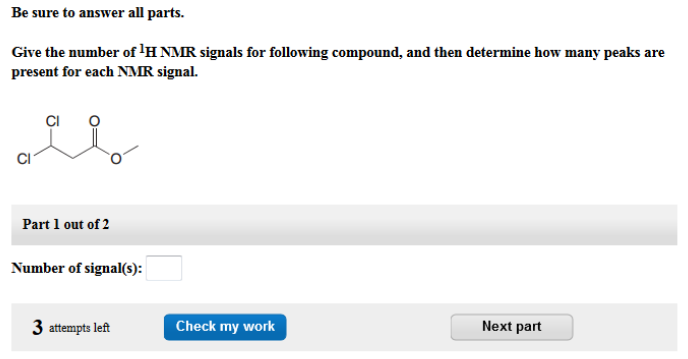# Problem: Be sure to answer all parts.Give the number of 1H NMR signals for following compound, and then determine how many peaks are present for each NMR signal. Number of signal(s):

###### FREE Expert Solution

There are 3 unique peaks for this molecule which is shown below:

89% (382 ratings)###### Problem Details

Be sure to answer all parts.

Give the number of 1H NMR signals for following compound, and then determine how many peaks are present for each NMR signal.

Number of signal(s):